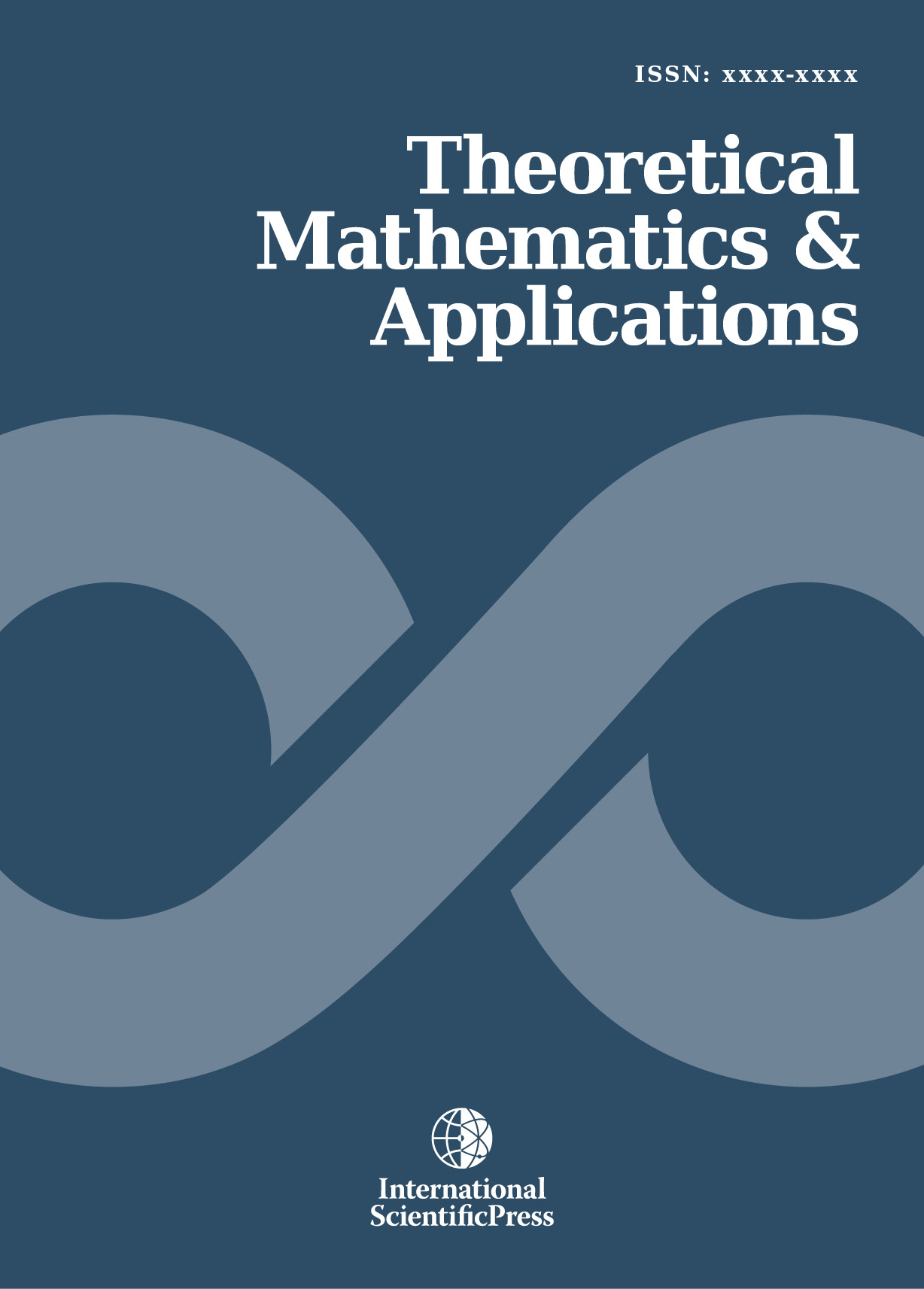# Theoretical Mathematics & Applications

#### Dynamics Classification of Boolean Networks and Solutions of Diophantine Equation

•[ Download ]
• Times downloaded: 1026
• Abstract

An effective scheme for coding Boolean networks is proposed, by which one can uniquely designate a distinguished integer in the range from 0 (to 2^n*2^n − 1) for any given n-node Boolean network. More importantly, by analyzing the characteristic polynomial of the linearized matrix of any given Boolean network, the connection between the dynamics of the network and the solution of a linear Diophantine equation can be established. Based on the calculation of the number of nonnegative integer solutions of the Diophantine equation, all n-node Boolean networks can be classified into several classes and the members in the same one have the same limit dynamical behaviors, i.e., same topological structures of invariant sets such as attractors and isles of Eden.ISSN: 1792-9687 (Print)
1792-9709 (Online)